Next: Test of the Initial Up: Restrictions on the Scenario Previous: Basic Observational Data for

## The COOC Criterion (Criterion of Observations versus Calculations)

In order to compare the number of different evolutionary stages obtained and X-ray luminosity of the chosen X-ray sources with the observed galactic values we use the sum of C/O+O/C, where C and O are the calculated and observed values of a given binary type, respectively. If we make comparison for a number of different binary species (for example, we compare the number of CV, number of X-ray pulsars,Ā total X-ray luminosity of LMXB, etc.), we write this criterion in the form:

Ā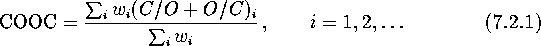where the sum is taken for each i-th species, and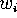are corresponding weights.

Obviously, this function reaches a minimum value of 2 once C=O for all species. When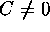at least for one of the species considered, this criterion rapidly increases (note that always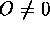by definition). This criterion is of course a rough measure of coincidence, and is used just to somehow compare the values which we know to within at least a factor of 2. We also note that the criterion in this form accounts, with equal power, for both rare encountered stages (for example, short-living superaccreting SS433-like binaries) and copious stages (for example, CV) of binary evolution.

As was noted by I.E.ĀPanchenko,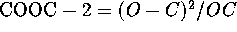, that is our criterion essentially represents a modification of the widely used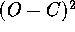value.

Mike E. Prokhorov
Sat Feb 22 18:38:13 MSK 1997